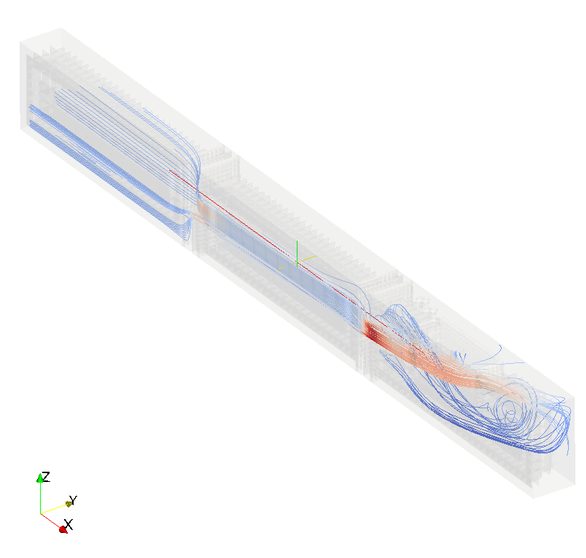# Simple decompression test case

Published: mer 14 janvier 2015

# Introduction

This test case aims to prove correlation for a simple three volumes rapid decompression.

Equations, and even physics, are not the same at all between 1D thermodynamical study and 3D CFD csimulation ; it would therefore be naive to expect an exact correlation between them. However on a simple case, it is very interesting and informative to correlate one against the other.

# Model

The model consists of three chained volumes of 3m³, connected via 0.5m² orifices.

The cabin parameters are defined as follows:

• $P = 1 bar$
• $T = 296 K (22.85 degC)$

The atmosphere is defined as follows:

• $P = 0.2 bar (alt=11.806 km)$
• $T = 216.66 K (-56.49 degC)$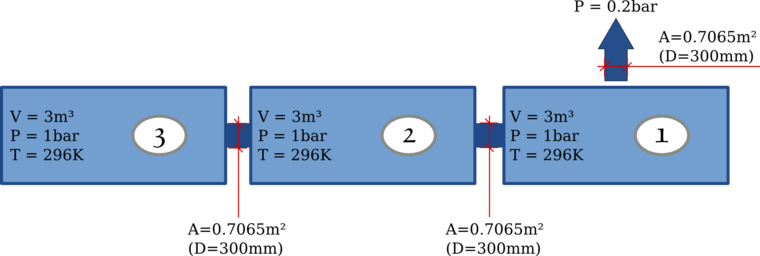# CFD study

The CFD study is performed with openFoam (http://www.openfoam.org/). The solver used is the transient sonic compressible solver called “sonicFoam”.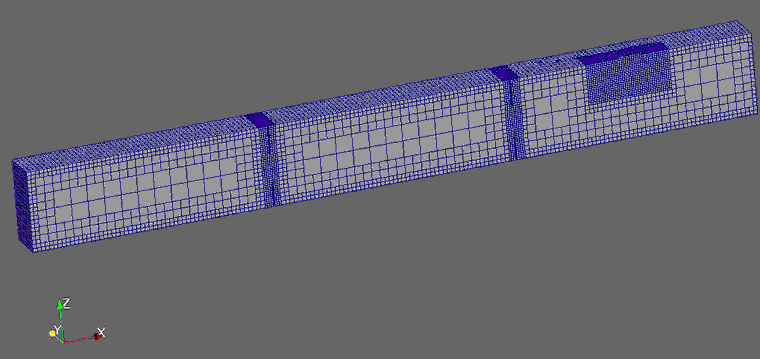# numeric internal decompression software

numeric‘s decompression program was run using the isentropic engine, based on the parameters described above.

As per common references, the discharge coefficient should be taken between 0.61 and 0.8. Two analysis were performed simulating two different discharge coefficients for internal vents.

# Compared Results

To extract values from the CFD results, two cells per volume (close to volume center) were selected and averaged.

## Correlations in pressure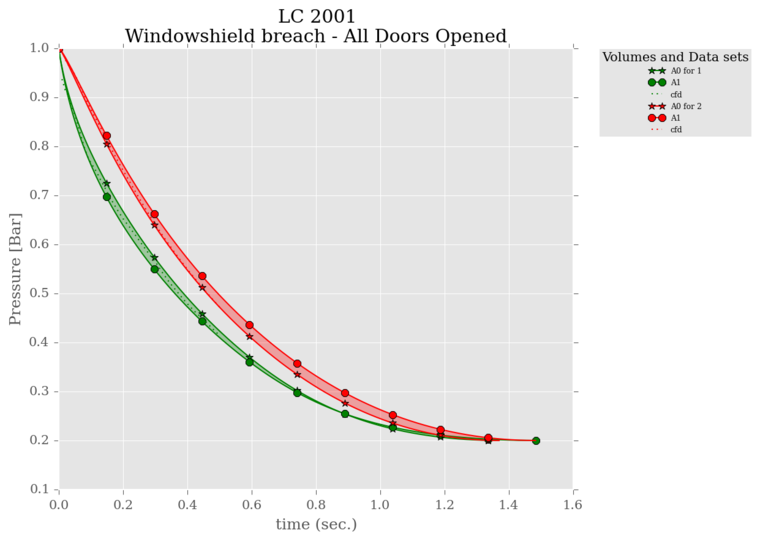Pressures from the CFD are clearly included inside the tiny pressures envelop given by the two analysis A0 and A1. CFD fits perfectly into it.

## DeltaP

The DeltaP are the most use full values for a decompression analysis: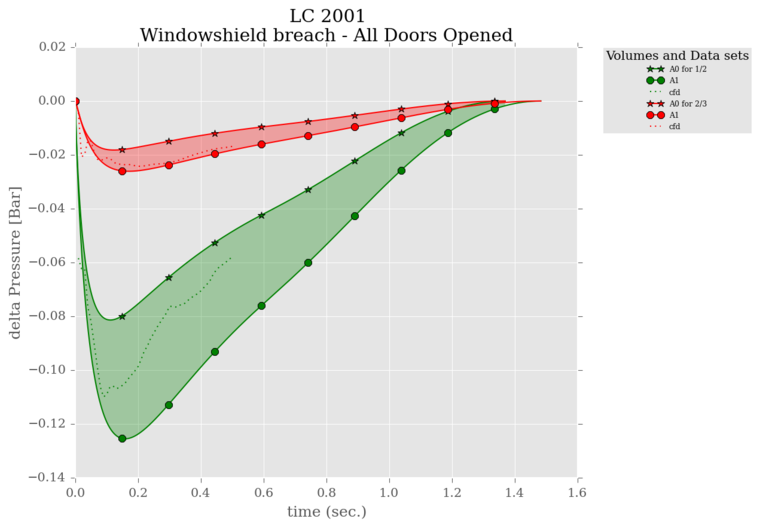## Correlations in temperature

Temperature are expected to correlate more badly, compared to pressure, since the unique parameter taken into account is the gas detent (thus pressure and mass), whereas the CFD will also take into account the calories transfer dur to massflows.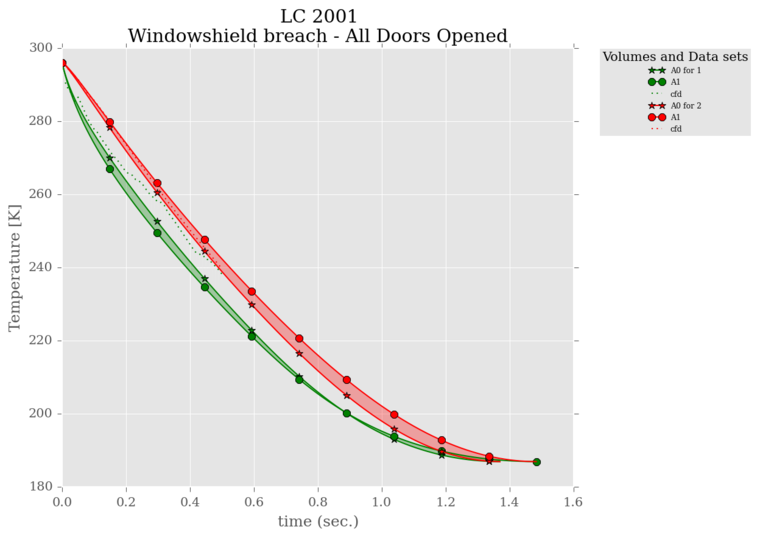# Conclusion

The correlations are really good with pressures using the regular Discharge Coefficients.